CE 338 2021 Spring
Lab 1: Superposition and Thevenin's Review
Nic Theobald
nstheobald@fortlewis.edu

Superposition and Thevenin's Review

### Introduction

This lab is a review of two circuit analysis methods, Superposition and Thevenin's equivalent circuits. Superposition is useful for when there are multiple voltage sources that can be turned on or off. Thevenin is useful for when a simplified version of the circuit is needed for further analysis.

## Methods and Materials

 Item Quantity LTspice 1

Superposition is the method of  solving a circuit while considering the affects of one voltage source at a time. The circuit in Figure 1 was solved by considering V1 and V2 independently. V2 was shorted and the voltages at each node were calculated, as well as the current through each resistor.
The same voltages and currents were then calculated when V1 was shorted. The voltages and currents were then summed to find the resulting voltages and currents. The second example first found the thevenin equyivalent of the original circuit. The thevenin equivalent was then used to find a reasonable on/off tme for the RC circuit.

## Results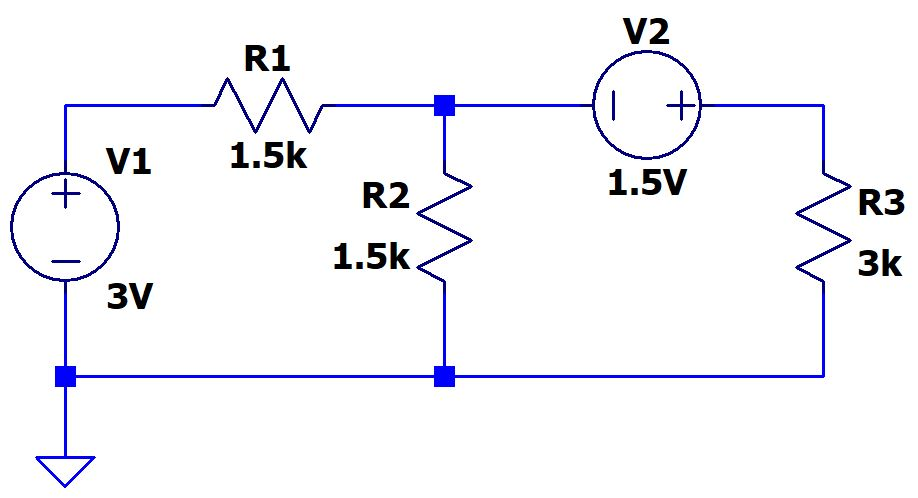Figure 1: Original Circuit
First, the circuit was solved when V2 was shorted.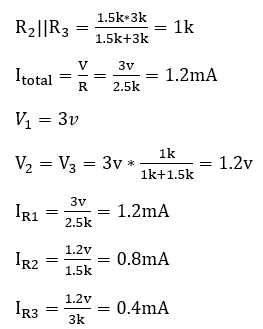Then the circuit was solved when V1 was shorted.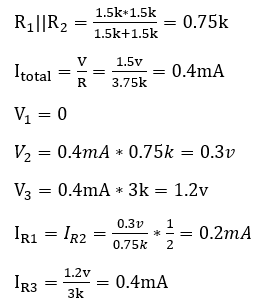Currents and Voltages were then summed.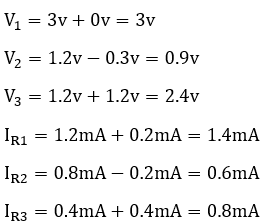s

The circuit was then simulated in LTspice.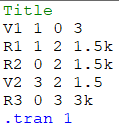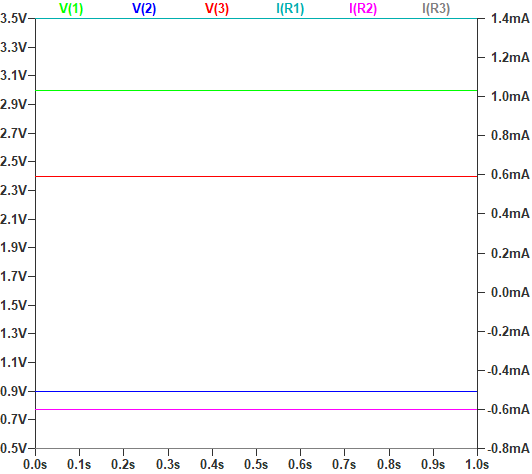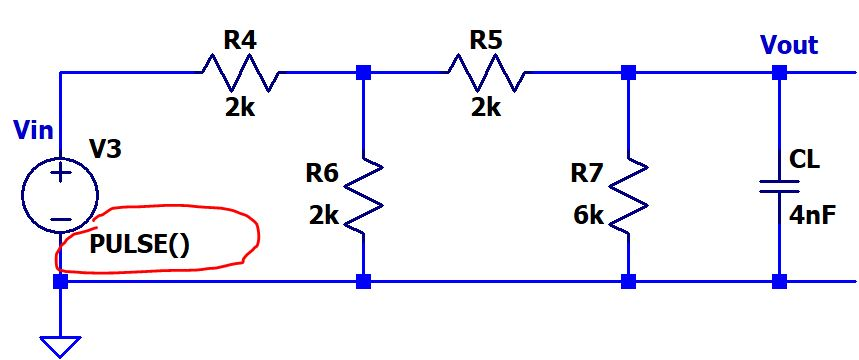First, V1 was shorted and Rth was found.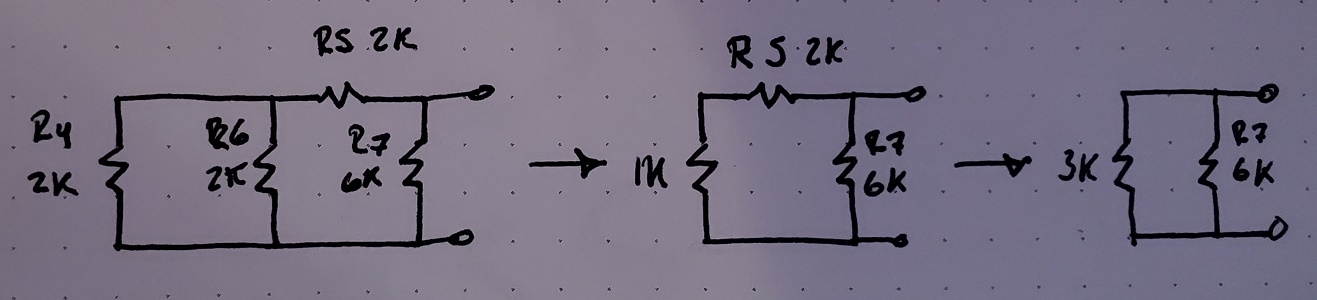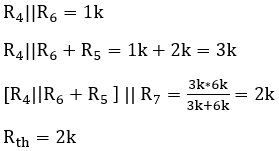Then Eth was found.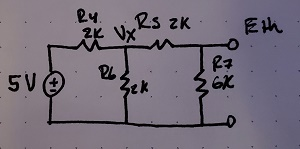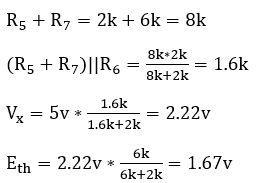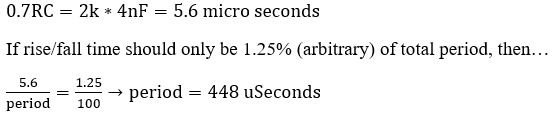Then the circuit was simulated.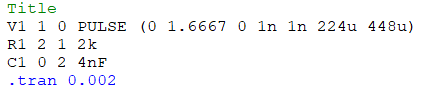### Discussion:

This lab reviewed Superposition and Thevenin equivalent crcuits. The first example was a simple circuit easily solved by superposition. The second example required the circuits Thevenin equivalent to determine a reasonable pulse on/off time.